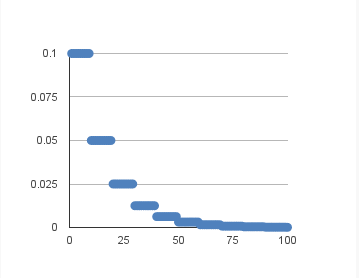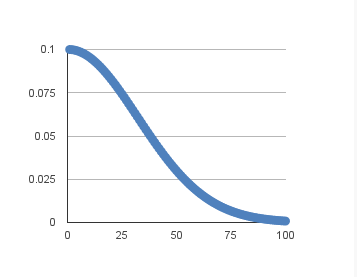• ## keras使用

千次阅读 2017-04-12 21:27:55
1数据...来自http://blog.csdn.net/u012162613/article/details/453970332 keras使用（jupyter notebook）参考： https://elitedatascience.com/keras-tutorial-deep-learning-in-python#step-4mni
1数据

http://pan.baidu.com/s/1qCdS6
来自http://blog.csdn.net/u012162613/article/details/45397033

2 keras使用（jupyter notebook）

参考： https://elitedatascience.com/keras-tutorial-deep-learning-in-python#step-4

mnist_floder = '/home/hx133330/data/mnist'

import os
import numpy as np
import cv2
from PIL import Image

len(os.listdir(mnist_floder))

42000


data = np.empty((42000,1,28,28),dtype = np.float32)

label = np.empty((42000,),dtype = np.uint8)

imgs = os.listdir(mnist_floder)

imgs

'5.552.jpg'


for i in range(len(imgs)):
data[i,:,:,:] = img
label[i] = int(imgs[i].split('.'))

data.shape

(42000, 1, 28, 28)


print label[0:10]

[5 7 7 0 5 3 2 6 3 1]


from matplotlib import pyplot as plt
plt.imshow(data[0,0,:,:])
plt.show()data /= 255

from keras.utils import np_utils
label = np_utils.to_categorical(label, 10)

label

array([ 0.,  0.,  0.,  0.,  0.,  1.,  0.,  0.,  0.,  0.])


from keras.models import Sequential
from keras.layers import Dense, Dropout, Activation, Flatten
from keras.layers import Convolution2D, MaxPooling2D

help(Convolution2D)

Help on class Convolution2D in module keras.layers.convolutional:

class Convolution2D(keras.engine.topology.Layer)


…

X_train = data.reshape(data.shape, 28, 28, 1)

model = Sequential()
model.add(Convolution2D(32, 3, 3, activation='relu', input_shape=(28,28,1)))

print model.output_shape

(None, 26, 26, 32)


model.add(Convolution2D(32, 3, 3, activation='relu'))
model.add(Dropout(0.25))

print model.output_shape

(None, 12, 12, 32)


model.add(Flatten())

print model.output_shape

(None, 4608)


model.add(Dense(128, activation='relu'))

print model.output_shape

(None, 128)


model.add(Dropout(0.5))
model.add(Dense(10, activation='softmax'))

print model.output_shape

(None, 10)


model.compile(loss='categorical_crossentropy',
metrics=['accuracy'])

model.fit(X_train, label,
batch_size=32, nb_epoch=10, verbose=1)

Epoch 1/10
16960/42000 [===========>..................] - ETA: 19s - loss: 0.4346 - acc: 0.8631

展开全文• keras使用tensorflow作为后端运行的话，tensorflow是自动识别设备 以下是强制使用CPU或GPU设备。 import tensorflow as tf sess = tf.Session(config=tf.ConfigProto(device_count={'gpu':0})) import tensorflow ...
设置GPU计算
keras使用tensorflow作为后端运行的话，tensorflow是自动识别设备
以下是强制使用CPU或GPU设备。
import tensorflow as tf
sess = tf.Session(config=tf.ConfigProto(device_count={'gpu':0}))

或者
import tensorflow as tf
import keras.backend.tensorflow_backend as KTF
KTF.set_session(tf.Session(config=tf.ConfigProto(device_count={'gpu':0})))



展开全文• Keras使用center-losss损失函数\Keras自定义损失函数
目录1、站在巨人的肩膀上2、Keras的损失函数3、在Keras实现center-loss损失函数3.1、导入库和定义常量3.2、实现多元分类softmax损失函数3.3、实现center-loss损失函数3.4、把softmax-loss和center-loss相加4、在模型中使用新的损失函数5、完整代码
1、站在巨人的肩膀上

TensorFlow实现center loss。
损失函数改进之Center Loss。

2、Keras的损失函数
想必大家都知道编译（model.compile）模型时，需要指定loss这个参数，也就是损失函数。实际上，Keras已经实现了很多损失函数，比如：
# 多元分类（分好多个类）损失函数
def categorical_crossentropy(y_true, y_pred):
return K.categorical_crossentropy(y_true, y_pred)
# 稀疏多元分类损失函数
def sparse_categorical_crossentropy(y_true, y_pred):
return K.sparse_categorical_crossentropy(y_true, y_pred)
# 二元分类损失函数
def binary_crossentropy(y_true, y_pred):
return K.mean(K.binary_crossentropy(y_true, y_pred), axis=-1)

观察上面的损失函数，参数都是y_true和y_pred。y_true是使用one_hot编码之后的标签，shape为(batch_size，NUM_CLASSES)；y_pred是模型最后一层（一般为softmax层）的输出，shape为(batch_size，NUM_CLASSES)；损失函数返回的shape为(batch_size，)。其中NUM_CLASSES是在多元分类问题中类别的数量。
因此，想要自定义损失函数，也非常简单，只要定义一个函数类似于：
def my_loss(y_true, y_pred):
m_loss=...
return m_loss

3、在Keras实现center-loss损失函数
三步走！第一步是去掉原模型最后的一层softmax层，直接获取最后一层fc层的输出，因为center-loss需要获取fc层的输出作为输入。第二步是实现多元分类softmax损失函数。第三步就是实现center-loss损失函数。还有附加的一步，就是把第二部的softmax损失加上第三步的center-loss损失。
因为我的keras是使用tensorflow作为后端，所以可以使用tensorflow的代码来实现损失函数。另外，为了实现代码的移植性，推荐使用keras.backend里面的方法来实现损失函数。 第3部分的代码在cl.py中实现。
3.1、导入库和定义常量
去掉模型的softmax层很简单，就不多说了。接下来是导入库和定义常量：
# coding=utf-8
# cl.py
# 兼容python3
from __future__ import absolute_import
from __future__ import division
from __future__ import print_function

from tensorflow import keras
from tensorflow.python.keras import backend as K
import tensorflow as tf
import numpy as np
import random

# 分类问题的类数，fc层的输出单元个数
NUM_CLASSES = 240
# 更新中心的学习率
ALPHA = 0.6
# center-loss的系数
LAMBDA = 0.0005

ALPHA是更新中心的学习率，一般设置为0.6或者0.5。LAMBDA是文中center-loss项的系数，这个参数需要仔细的调教，才能取得很好的效果。
3.2、实现多元分类softmax损失函数
这里说的多元分类softmax损失函数就是categorical_crossentropy（多类别分类的损失函数，标签需要使用one_hot编码）。非常简单，如下：
def softmax_loss(labels, features):
"""
计算softmax-loss
:param labels: 等同于y_true，使用了one_hot编码，shape应为(batch_size, NUM_CLASSES)
:param features: 等同于y_pred，模型的最后一个fc层(不是softmax层)的输出，shape应为(batch_size, NUM_CLASSES)
:return: 多云分类的softmax-loss损失，shape为(batch_size, )
"""
return K.categorical_crossentropy(labels, K.softmax(features, axis=-1))

这里为什么要重新实现而不直接使用keras.loss.categorical_crossentropy呢？因为去掉了模型的softmax层，这里要对模型最后一层的输出，也就是features，调用softmax处理一遍，再作为K.categorical_crossentropy的参数。
3.3、实现center-loss损失函数
我在这里借鉴了另外一位大佬为tensorflow实现的center-loss代码，因为我的keras使用tensorflow作为后端，所以按道理是可以直接用的。先给出计算center-loss的代码：
def center_loss(labels, features, alpha=_g.ALPHA, num_classes=_g.NUM_CLASSES):
"""
获取center loss及更新样本的center
:param labels: Tensor,表征样本label,非one-hot编码,shape应为(batch_size,).
:param features: Tensor,表征样本特征,最后一个fc层的输出,shape应该为(batch_size, num_classes).
:param alpha: 0-1之间的数字,控制样本类别中心的学习率,细节参考原文.
:param num_classes: 整数,表明总共有多少个类别,网络分类输出有多少个神经元这里就取多少.
:return: Tensor, center-loss， shape因为(batch_size,)
"""
# 获取特征的维数，例如256维
len_features = features.get_shape()
# 建立一个Variable,shape为[num_classes, len_features]，用于存储整个网络的样本中心，
# 设置trainable=False是因为样本中心不是由梯度进行更新的
centers = tf.get_variable('centers', [num_classes, len_features], dtype=tf.float32,
initializer=tf.constant_initializer(0), trainable=False)
# 将label展开为一维的，如果labels已经是一维的，则该动作其实无必要
labels = tf.reshape(labels, [-1])

# 根据样本label,获取mini-batch中每一个样本对应的中心值
centers_batch = tf.gather(centers, labels)

# 当前mini-batch的特征值与它们对应的中心值之间的差
diff = centers_batch - features

# 获取mini-batch中同一类别样本出现的次数,了解原理请参考原文公式(4)
unique_label, unique_idx, unique_count = tf.unique_with_counts(labels)
appear_times = tf.gather(unique_count, unique_idx)
appear_times = tf.reshape(appear_times, [-1, 1])

diff = diff / tf.cast((1 + appear_times), tf.float32)
diff = alpha * diff

# 更新centers
centers_update_op = tf.scatter_sub(centers, labels, diff)

# 这里使用tf.control_dependencies更新centers
with tf.control_dependencies([centers_update_op]):
# 计算center-loss
c_loss = tf.nn.l2_loss(features - centers_batch)

return c_loss

这里说一下我当时遇到的难题，因为原代码中，是返回c_loss、centers和centers_update_op，然后使用sess.run来更新centers，但损失函数只能返回损失项c_loss，并不能返回centers_update_op，也就不能更新centers。最后想到的办法是使用tf.control_dependencies强行在计算c_loss之前更新centers，这样，centers就会更新了。
3.4、把softmax-loss和center-loss相加
def loss(labels, features):
"""
使用这个函数来作为损失函数，计算softmax-loss加上一定比例的center-loss
:param labels: Tensor，等同于y_true，使用了one_hot编码，shape应为(batch_size, NUM_CLASSES)
:param features: Tensor， 等同于y_pred, 模型的最后一个fc层(不是softmax层)的输出，shape应为(batch_size, NUM_CLASSES)
:return: softmax-loss加上一定比例的center-loss
"""
labels = K.cast(labels, dtype=tf.float32)
# 计算softmax-loss
sf_loss = softmax_loss(labels, features)
# 计算center-loss，因为labels使用了one_hot来编码，所以这里要使用argmax还原到原来的标签
c_loss = center_loss(K.argmax(labels, axis=-1), features)
return sf_loss + LAMBDA * c_loss

LAMBDA这个是一个需要仔细调教的参数，对于我的模型，不同的大小对模型训练的效果影响比较大。测试新的softmax-loss with center-loss：
if __name__ == '__main__':
# 测试label和测试用的features
test_features = np.random.randn(32, NUM_CLASSES).astype(dtype=np.float32)
test_labels = np.array(random.sample(range(0, NUM_CLASSES - 1), 32))
test_labels = 0
# one_hot编码
test_labels = keras.utils.to_categorical(test_labels, NUM_CLASSES)

print(test_features.shape, test_labels.shape)

# 新建tensor
test_features = tf.constant(test_features)
test_labels = tf.constant(test_labels)
# 得到计算损失的op
loss_op = loss(test_labels, test_features)
with tf.Session() as sess:
# 初始化变量
sess.run(tf.global_variables_initializer())
# 计算损失
result = sess.run(loss_op)
print(result.shape)
print(result)
# 把centers取出来，看看有没有更新
centers = sess.graph.get_tensor_by_name('centers:0')
print(centers.eval().shape)
print(centers.eval())

4、在模型中使用新的损失函数
未使用新的损失函数前，模型的最后一层是softmax层，编译模型和训练模型的部分代码如下：
    # 创建训练集，train_dataset是tf.data.Dataset实例，train_steps是训练一遍需要的步数
train_dataset, train_steps = ...
# 创建验证集
val_dataset, val_steps = ...
# 获取模型
model = ...
# 编译模型
loss=keras.losses.categorical_crossentropy, # 损失函数
metrics=[keras.metrics.categorical_accuracy]) # 指标
# 训练模型
model.fit(train_dataset, epochs=10, steps_per_epoch=train_steps,
validation_data=val_dataset, validation_steps=val_steps)

使用center-loss之后，编译和训练模型的代码如下：
    import cl

# 创建训练集，train_dataset是tf.data.Dataset实例，train_steps是训练一遍需要的步数
train_dataset, train_steps = ...
# 创建验证集
val_dataset, val_steps = ...
# 获取模型
model = ...
# 编译模型
loss=cl.loss, # 损失函数
metrics=[cl.categorical_accuracy]) # 指标
# 在这里初始化变量
sess = K.get_session()
sess.run(tf.global_variables_initializer())
# 训练模型
model.fit(train_dataset, epochs=10, steps_per_epoch=train_steps,
validation_data=val_dataset, validation_steps=val_steps)

特别需要注意的是，一定要在编译（compile）模型之后，训练（fit）或者评估（evaluate）之前，使用tf.global_variables_initializer初始化center-loss函数中定义的centers，否则会报错说试图使用未初始化的变量。
观察上面的代码，指标后面的函数cl.categorical_accuracy也是重新实现在cl.py中的：
def categorical_accuracy(y_true, y_pred):
"""
重写categorical_accuracy函数，以适应去掉softmax层的模型
:param y_true: 等同于labels，
:param y_pred: 等同于features。
:return: 准确率
"""
# 计算y_pred的softmax值
sm_y_pred = K.softmax(y_pred, axis=-1)
# 返回准确率
return K.cast(K.equal(K.argmax(y_true, axis=-1), K.argmax(sm_y_pred, axis=-1)), K.floatx())

和原来keras.metrics.categorical_accuracy相比，因为去掉了模型最后的softmax层，所以需要softmax函数处理一下y_pred。
5、完整代码
为方便大家，贴上完整代码：
# coding=utf-8
# 兼容python3
from __future__ import absolute_import
from __future__ import division
from __future__ import print_function

from tensorflow import keras
from tensorflow.python.keras import backend as K
import tensorflow as tf
import numpy as np
import random

# 分类问题的类数，fc层的输出单元个数
NUM_CLASSES = 240
# 更新中心的学习率
ALPHA = 0.6
# center-loss的系数
LAMBDA = 0.0005

def center_loss(labels, features, alpha=ALPHA, num_classes=NUM_CLASSES):
"""
获取center loss及更新样本的center
:param labels: Tensor,表征样本label,非one-hot编码,shape应为(batch_size,).
:param features: Tensor,表征样本特征,最后一个fc层的输出,shape应该为(batch_size, num_classes).
:param alpha: 0-1之间的数字,控制样本类别中心的学习率,细节参考原文.
:param num_classes: 整数,表明总共有多少个类别,网络分类输出有多少个神经元这里就取多少.
:return: Tensor, center-loss， shape因为(batch_size,)
"""
# 获取特征的维数，例如256维
len_features = features.get_shape()
# 建立一个Variable,shape为[num_classes, len_features]，用于存储整个网络的样本中心，
# 设置trainable=False是因为样本中心不是由梯度进行更新的
centers = tf.get_variable('centers', [num_classes, len_features], dtype=tf.float32,
initializer=tf.constant_initializer(0), trainable=False)
# 将label展开为一维的，如果labels已经是一维的，则该动作其实无必要
labels = tf.reshape(labels, [-1])

# 根据样本label,获取mini-batch中每一个样本对应的中心值
centers_batch = tf.gather(centers, labels)

# 当前mini-batch的特征值与它们对应的中心值之间的差
diff = centers_batch - features

# 获取mini-batch中同一类别样本出现的次数,了解原理请参考原文公式(4)
unique_label, unique_idx, unique_count = tf.unique_with_counts(labels)
appear_times = tf.gather(unique_count, unique_idx)
appear_times = tf.reshape(appear_times, [-1, 1])

diff = diff / tf.cast((1 + appear_times), tf.float32)
diff = alpha * diff

# 更新centers
centers_update_op = tf.scatter_sub(centers, labels, diff)

# 这里使用tf.control_dependencies更新centers
with tf.control_dependencies([centers_update_op]):
# 计算center-loss
c_loss = tf.nn.l2_loss(features - centers_batch)

return c_loss

def softmax_loss(labels, features):
"""
计算softmax-loss
:param labels: 等同于y_true，使用了one_hot编码，shape应为(batch_size, NUM_CLASSES)
:param features: 等同于y_pred，模型的最后一个FC层(不是softmax层)的输出，shape应为(batch_size, NUM_CLASSES)
:return: 多云分类的softmax-loss损失，shape为(batch_size, )
"""
return K.categorical_crossentropy(labels, K.softmax(features, axis=-1))

def loss(labels, features):
"""
使用这个函数来作为损失函数，计算softmax-loss加上一定比例的center-loss
:param labels: Tensor，等同于y_true，使用了one_hot编码，shape应为(batch_size, NUM_CLASSES)
:param features: Tensor， 等同于y_pred, 模型的最后一个fc层(不是softmax层)的输出，shape应为(batch_size, NUM_CLASSES)
:return: softmax-loss加上一定比例的center-loss
"""
labels = K.cast(labels, dtype=tf.float32)
# 计算softmax-loss
sf_loss = softmax_loss(labels, features)
# 计算center-loss，因为labels使用了one_hot来编码，所以这里要使用argmax还原到原来的标签
c_loss = center_loss(K.argmax(labels, axis=-1), features)
return sf_loss + LAMBDA * c_loss

def categorical_accuracy(y_true, y_pred):
"""
重写categorical_accuracy函数，以适应去掉softmax层的模型
:param y_true: 等同于labels，
:param y_pred: 等同于features。
:return: 准确率
"""
# 计算y_pred的softmax值
sm_y_pred = K.softmax(y_pred, axis=-1)
# 返回准确率
return K.cast(K.equal(K.argmax(y_true, axis=-1), K.argmax(sm_y_pred, axis=-1)), K.floatx())

if __name__ == '__main__':
# 测试label和测试用的features
test_features = np.random.randn(32, NUM_CLASSES).astype(dtype=np.float32)
test_labels = np.array(random.sample(range(0, NUM_CLASSES - 1), 32))
test_labels = 0
# one_hot编码
test_labels = keras.utils.to_categorical(test_labels, NUM_CLASSES)

print(test_features.shape, test_labels.shape)

# 新建tensor
test_features = tf.constant(test_features)
test_labels = tf.constant(test_labels)
# 得到计算损失的op
loss_op = loss(test_labels, test_features)
with tf.Session() as sess:
# 初始化变量
sess.run(tf.global_variables_initializer())
# 计算损失
result = sess.run(loss_op)
print(result.shape)
print(result)
# 把centers取出来，看看有没有更新
updated_centers = sess.graph.get_tensor_by_name('centers:0')
print(updated_centers.eval().shape)
print(updated_centers.eval())




展开全文• ## Keras使用一些技巧

千次阅读 2018-01-31 10:33:17
我都一直在使用Keras框架，很多时候只是个‘搬砖’的，所以有必要学习一下keras使用技巧。


最近几个月为了写小论文，题目是关于用深度学习做人脸检索的，所以需要选择一款合适的深度学习框架，caffe我学完以后感觉使用不是很方便，之后有人向我推荐了Keras，其简单的风格吸引了我，之后的四个月我都一直在使用Keras框架，由于我用的时候，tensorflow的相关教程还不是很多，所以后端我使用theano。这次的心得主要分成两篇，第一篇是聊聊开始使用keras容易遇到的坑，第二篇会在我论文发了之后，连同代码和一些更细节的东西一起发出来，我也会在结尾放出一些自己收集的CNN和tensorflow的相关教程，就这样。

还有Keras的QQ群号：119427073
本文由浅入深聊一聊我在这个过程中遇到的一些问题：
1、Keras输出的loss，val这些值如何保存到文本中去：
Keras中的fit函数会返回一个History对象，它的History.history属性会把之前的那些值全保存在里面，如果有验证集的话，也包含了验证集的这些指标变化情况，具体写法：
hist=model.fit(train_set_x,train_set_y,batch_size=256,shuffle=True,nb_epoch=nb_epoch,validation_split=0.1)
with open('log_sgd_big_32.txt','w') as f:
f.write(str(hist.history))
我觉得保存之前的loss，val这些值还是比较重要的，在之后的调参过程中有时候还是需要之前loss的结果作为参考的，特别是你自己添加了一些自己的loss的情况下，但是这样的写法会使整个文本的取名比较乱，所以其实可以考虑使用Aetros的插件，Aetros网址，这是一个基于Keras的一个管理工具，可以可视化你的网络结构，中间卷积结果的可视化，以及保存你以往跑的所有结果，还是很方便的，就是有些不稳定，有时候会崩。。。
2、关于训练集，验证集和测试集：
其实一开始我也没搞清楚这个问题，拿着测试集当验证集用，其实验证集是从训练集中抽取出来用于调参的，而测试集是和训练集无交集的，用于测试所选参数用于该模型的效果的，这个还是不要弄错了。。。在Keras中，验证集的划分只要在fit函数里设置validation_split的值就好了，这个对应了取训练集中百分之几的数据出来当做验证集。但由于shuffle是在validation _split之后执行的，所以如果一开始训练集没有shuffle的话，有可能使验证集全是负样本。测试集的使用只要在evaluate函数里设置就好了。
print model.evaluate（test_set_x，test_set_y ,batch_size=256）
这里注意evaluate和fit函数的默认batch_size都是32，自己记得修改。
3、关于优化方法使用的问题：

有很多初学者人会好奇怎么使sgd的学习率动态的变化，其实Keras里有个反馈函数叫LearningRateScheduler，具体使用如下：
def step_decay(epoch):
initial_lrate = 0.01
drop = 0.5
epochs_drop = 10.0
lrate = initial_lrate * math.pow(drop,math.floor((1+epoch)/epochs_drop))
return lrate
lrate = LearningRateScheduler(step_decay)
sgd = SGD(lr=0.0, momentum=0.9, decay=0.0, nesterov=False)
model.fit(train_set_x, train_set_y, validation_split=0.1, nb_epoch=200, batch_size=256, callbacks=[lrate])
上面代码是使学习率指数下降，具体如下图：当然也可以直接在sgd声明函数中修改参数来直接修改学习率，学习率变化如下图：
sgd = SGD(lr=learning_rate, decay=learning_rate/nb_epoch, momentum=0.9, nesterov=True)1具体可以参考这篇文章Using Learning Rate Schedules for Deep Learning Models in Python with Keras
4、关于过拟合问题的讨论：
我现在所知道的解决方法大致只有两种，第一种就是添加dropout层，dropout的原理我就不多说了，主要说一些它的用法，dropout可以放在很多类层的后面，用来抑制过拟合现象，常见的可以直接放在Dense层后面，对于在Convolutional和Maxpooling层中dropout应该放置在Convolutional和Maxpooling之间，还是Maxpooling后面的说法，我的建议是试！这两种放置方法我都见过，但是孰优孰劣我也不好说，但是大部分见到的都是放在Convolutional和Maxpooling之间。关于Dropout参数的选择，这也是只能不断去试，但是我发现一个问题，在Dropout设置0.5以上时，会有验证集精度普遍高于训练集精度的现象发生，但是对验证集精度并没有太大影响，相反结果却不错，我的解释是Dropout相当于Ensemble，dropout过大相当于多个模型的结合，一些差模型会拉低训练集的精度。当然，这也只是我的猜测，大家有好的解释，不妨留言讨论一下。

当然还有第二种就是使用参数正则化，也就是在一些层的声明中加入L1或L2正则化系数，正则化的原理什么的我就不细说了，具体看代码：
C1 = Convolution2D(20, 4, 4, border_mode='valid', init='he_uniform', activation='relu',W_regularizer=l2(regularizer_params))1其中W_regularizer=l2(regularizer_params)就是用于设置正则化的系数，这个对于过拟合有着不错的效果，在一定程度上提升了模型的泛化能力。
5、Batchnormalization层的放置问题：
BN层是真的吊，简直神器，除了会使网络搭建的时间和每个epoch的时间延长一点之外，但是关于这个问题我看到了无数的说法，对于卷积和池化层的放法，又说放中间的，也有说池化层后面的，对于dropout层，有说放在它后面的，也有说放在它前面的，对于这个问题我的说法还是试！虽然麻烦。。。但是DL本来不就是一个偏工程性的学科吗。。。还有一点是需要注意的，就是BN层的参数问题，我一开始也没有注意到，仔细看BN层的参数：
keras.layers.normalization.BatchNormalization(epsilon=1e-06, mode=0, axis=-1, momentum=0.9, weights=None, beta_init='zero', gamma_init='one')

mode：整数，指定规范化的模式，取0或1
0：按特征规范化，输入的各个特征图将独立被规范化。规范化的轴由参数axis指定。注意，如果输入是形如（samples，channels，rows，cols）的4D图像张量，则应设置规范化的轴为1，即沿着通道轴规范化。输入格式是‘tf’同理。
1：按样本规范化，该模式默认输入为2D
我们大都使用的都是mode=0也就是按特征规范化，对于放置在卷积和池化之间或之后的4D张量，需要设置axis=1，而Dense层之后的BN层则直接使用默认值就好了。
总结
这次暂时先写这么多，这次写的比较浅，还有很多拓展的东西都下次再写了，下面给大家附上一些不错的资料吧~
CNN基础：
【1】你真的了解CNN网络吗？

【2】CNN近期发展和实用技巧（上）

【3】深度
| 从入门到精通：卷积神经网络初学者指南（附论文）
【4】Convolutional Neural Networks (CNNs): An Illustrated Explanation

【5】Convolutional Neural Networks backpropagation: from intuition to derivation

【6】贺完结！CS231n官方笔记授权翻译总集篇发布
DL和Keras相关：
【1】深度学习中的激活函数导引

【2】深度网络的过拟合问题讨论

【3】How To Improve Deep Learning Performance

【5】Keras/Python深度学习中的网格搜索超参数调优（附源码）

【6】Yoshua Bengio等大神传授：26条深度学习经验

【7】leriomaggio/deep-learning-keras-euroscipy2016
Tensorflow相关：
【1】TensorFlow深度学习，一篇文章就够了

【2】alrojo/tensorflow-tutorial

【3】莫烦 tensorflow 神经网络 教程

展开全文深度学习
• keras 使用self-attention 安装 pip install keras-self-attention 使用demo import keras from keras_self_attention import SeqSelfAttention model = keras.models.Sequential(...
• Keras使用陷阱这里归纳了Keras使用过程中的一些常见陷阱和解决方法，如果你的模型怎么调都搞不对，或许你有必要看看是不是掉进了哪个猎人的陷阱，成为了一只嗷嗷待宰（？）的猎物Keras陷阱不多，我们保持更新，希望...
• 深度学习之keras使用keras安装 安装Numpy、Scipy等科学计算库 安装theano、tensorflow eg:CPU版tensorflow pip install tensorflow pip install keras 修改Backend底层框架Theano或者Tensorflow使用import keras屏幕...深度学习
• Keras介绍 简介 Keras是更高层的深度学习框架，它可以运行在其它的底层框架上面，包括TensorFlow和CNTK。也就是说，keras内部会调用tensorflow来完成任务。换句话说，就是keras比tensorflow更加方便。 导入keras # ...tensorflow 神经网络
• 项目源代码：https://github.com/zhongqianli/learning_keras ...本文将介绍如何使用keras训练深度学习模型。 一、读取数据 （1）数据准备 本文使用手写体数字识别数据库mnist。keras通过mnist.load_data()即...
• ## keras使用Tensorboard

千次阅读 2018-09-19 15:56:28
tensorboard可視化 夠震撼，但是 使用tf來生成log，有點麻煩。 那就是用tf的高層封裝 Keras來生成log,進而啟動tensorboard。 只需要 引入 tensorBoard from keras.callbacks import TensorBoard, 然後在fit的...
• 深度学习框架Keras使用心得 https://blog.csdn.net/u010159842/article/details/54136177#commentBox   Keras使用的一些细节 https://www.cnblogs.com/ranjiewen/p/8011021.html
• pip install --upgrade keras==2.1.0 升级到指定版本 pip install keras==2.1.0 安装指定版本 查看版本 python &gt;&gt;&gt; import keras &gt;&gt;&gt; print keras.__version__ ...
• ## keras使用RNN

千次阅读 2019-08-18 00:57:27
文字感情分析 参考:...# 数据预处理 from keras.datasets import imdb from ke...机器学习
• keras 使用Albumentations库自定义数据增强器Sequence自定义数据增强器 Sequence keras.utils.Sequence() 用于拟合数据序列的基对象，例如一个数据集。 每一个 Sequence 必须实现 getitem 和 len 方法。 如果你想在...
• Keras 使用自己的数据分类，并使用tensorboard记录的简单实例实例
• [转]keras使用入门及3D神经网络资源 原文链接https://blog.csdn.net/lengxiaomo123/article/details/68926778 keras使用入门及3D卷积神经网络资源 keras模型 Sequential...
• Keras使用多张显卡训练还是比较方便，在代码中添加下面代码就可以 import os #指定显卡 os.environ["CUDA_VISIBLE_DEVICES"] = "4, 5" from keras.utils import multi_gpu_model #分解模型 parallel_model = ...
• 问题如下： 解决方案： 1，将keras包更新到最新； 2，运行install_keras()R 深度学习
• keras使用GPU训练： 1、简单方法：直接在运行前加　CUDA_VISIBLE_DEVICES=1 CUDA_VISIBLE_DEVICES=1 python train.py 2、指定一块或多块GPU import os os.environ["CUDA_DEVICE_ORDER"]="PCI_BUS_ID" ...keras
• 【背景】【WIN10系统。在只有一块GPU的情况下，为了能同时利用cpu和gpu跑keras】网上找了一堆帖子都不管用 【方法】 import os # Uncomment the line below to make ...【使用CPU跑keras的情况下输出】 【使...
• ## keras使用GPU

千次阅读 2017-10-18 17:21:37
验证是否启动GPU from theano import function, config, shared, sandbox import theano.tensor as T import numpy import time vlen = 10 * 30 * 768 # 10 x #cores x # threads per core ...
• Keras使用全神经网络(dense)将testaccrucy测试准确性多次调试后到达98.5%。1.原代码import tensorflow as tf import tensorflow.contrib.keras as keras from PIL import Image import numpy as np import ...全连接层
• 本人机子内存8G，显卡GTX1060 6G显存，用 keras 跑小例子时候也能跑，也没发现自己运行时到底是用的CPU还是GPU，这次跑了稍微长点的例子，然后 jupyter notebook 内核就死了，就想起来验证一下到底用的是什么，网上...
• 最近有机会蹭组里的图形工作站跑DNN，想着终于有机会感受一下GPU的速度，...keras使用CPU和GPU运算没有任何的语法差别，它能自动地判断能不能使用GPU运算，能的话就用GPU，不能则CPU。你只需要在代码开头加上下面这...教程 CUDA cuDNN GPU运算
• ## 深度学习框架Keras使用心得

万次阅读 多人点赞 2016-10-12 22:04:19
题目是关于用深度学习做人脸检索的，所以需要选择一款合适的深度学习框架，caffe我学完以后感觉使用不是很方便，之后有人向我推荐了Keras，其简单的风格吸引了我，之后的四个月我都一直在使用Keras框架，由于我用的...框架 深度学习 Tensorflow
• 本文主要介绍使用LSTM实现字符级文本生成。 下面是示例代码： # coding: utf-8 # In: # 下载语料库并将其转化为小写 import keras import numpy as np path = keras.utils.get_file( 'nietzsche.txt', ...
• 几乎所有相关代码都是backend里面，tensorflow与theano共同拥有的函数在backend.common里面，这部分代码无论使用任何...如果使用tensorflow backend，几乎所有的Keras.tensor都可以使用tensorflow内部的Operator。函数...